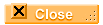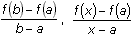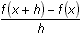AP Calculus - Glossary Roots A root, or zero, of a function is a value of the independent variable that makes the function zero, i.e., solutions to f(x) = 0 are the roots, or zeros of the function f.Guess and Check Guess and check is a method for finding roots (or zeros) of a function. First guess a value for the variable and find the value of the function. If the value of the function is not zero, pick another value of the variable and find the value of the function. Continue in this manner until a root is found.Viewing Window The viewing window represents the portion of the coordinate plane displayed on the Graph screen. By setting Window variables, you can define the viewing window's boundaries and other attributes. Each of the graphing modes (function, parametric, etc.) has it's own independent set of window variables.Logistic Function Logistic functions are used to represent growth that has a limiting factor, such as food supplies, war, new diseases, etc. Logistic models are often used to model population growth or the spread of disease or rumor.Regression Equation An equation that represents a set of data is called a regression equation, and it is used to estimate or predict values of a data set.Random Number A random number is a number chosen as though by chance in some specified range. The random number feature on the TI-89 can be used to generate a series of one or more random numbers.Scatter Plot A scatter plot is a graphical display of data plotted as points on a coordinate plane to show the relationship between two quantities.Random Seed The random seed is a number stored in the calculator which determines what random number will be generated next. When a random number is generated, the seed is changed so that the next random number will be different. However, two calculators starting with the same random seed will generate identical sequence of random numbers. The random seed is set to 0 at the factory, so in order to ensure that each student will generate a different sequence of random numbers, it's necessary to set the random seed to different values in each of their calculators.Residual A residual is a measure of the error between an actual data value and the corresponding value given by an equation. That is, the residual is d – y where (x, d) is the data point and (x, y) is the point determined by the equation.Derivative The derivative of a function is an expression that represents the instantaneous rate of change of the value of the function relative to changes in the input variable.Concave Upward A curve is concave upward if the curve lies above the tangents.Concave Downward A curve is concave downward if the curve lies below the tangents.Inflection Point A point where the graph of a function changes concavity is called an inflection point.Semimajor Axis A semimajor axis of an ellipse or hyperbola is the line segment from the conic section's center to a vertex. The length of a semimajor axis is half the length of the major axis.Compound Inequality A compound (or extended) inequality is an inequality that compares more than two quantities and contains more than one inequality symbol.Oblique An oblique, or slanted, asymptote is a nonvertical and nonhorizontal line that the graph of a function approaches as the input gets large without bound.Proper Fraction A proper fraction is a rational expression in which the degree of the numerator is less than the degree of the denominator. An improper fraction is a rational expression in which the degree of the numerator is greater than or equal to the degree of the denominator. An improper fraction may be changed into a polynomial plus a proper fraction by dividing the numerator by the denominator. Any remainder is written as the numerator of a proper fraction whose denominator is the divisor.Tangent A tangent line to the graph of f at the point (a, f(a)) is the line through (a, f(a)) with slope, provided that the limit exists.Difference Quotients Expressions of the formandare called difference quotients because each is the quotient of two differences.Secant Line A secant line is a line through two points on the curve.Point-Slope Form for the Equation of a Line The equation of the line through the point (x1, y1) with slope m is y – y1 = m(x – x1).Differentiable A function is differentiable at a point if its derivative exists there.Turning Point A turning point of the graph of a function is a point where the function changes from increasing to decreasing or changes from decreasing to increasing.ncontour The ncontour window variable has no effect when the graph style is Implicit Plot.knot A knot is a unit used to measure the speed of a ship. One knot represents one nautical mile (6,076.1 feet) an hour.sum function The sum functionevaluates an expression at discrete variable values within a range and returns their sum. Its syntax is(expression, var, initial value, ending value).Midpoint Riemann sums Midpoint Riemann sums are obtained by using the midpoint of each subinterval on the x-axis to determine the height of the corresponding rectangle.Integral Function The syntax for finding a definite integral using the integral function is(expression, var, lower, upper)Absolute Value Absolute Value (abs) is found in the catalog.Factorial The factorial of a whole number is the product of the series of consecutive whole numbers that begin with 1 and end with the number: 6! = 1x2x3x4x5x6. 0! is defined to be 1.Scalar A scalar is a real number. It has magnitude but no direction.Rationalizing (a denominator): A fraction is rationalized by writing it as an equivalent fraction with no radicals in the denominator.©Copyright 2002 All rights reserved. | Trademarks | Privacy Policy | Link Policy